# Can We Engineer Clean Drinking Water?

### Are You Up for the Challenge?

Calculating Your Uncertainty Budget in Manufacturing
Jody Muelaner posted on October 09, 2017 |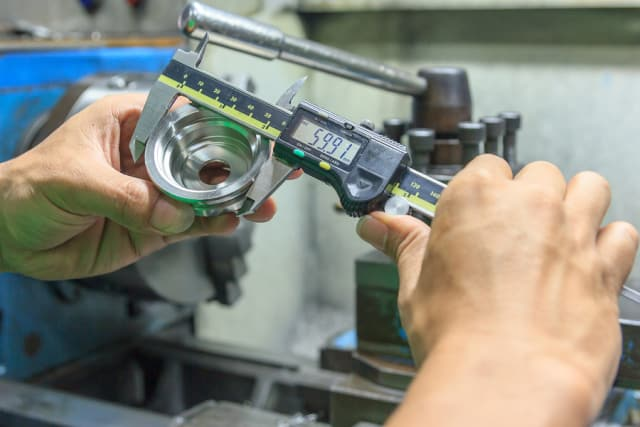This article follows on from my previous article ‘An Introduction to Metrology and Quality in Manufacturing’ which explains the fundamental concept of uncertainty. If you haven’t read that yet, I suggest you check it out first.

In this article, I will go into the details of how you actually calculate the uncertainty of a measurement.

## Sources of Uncertainty

My previous article introduced the idea that all measurements have uncertainty. This uncertainty stems from different sources, including repeatability, calibration and the environment. To find the uncertainty of a measurement result, we must first estimate the contribution from each source and then calculate the combined uncertainty.

The Guide to the Expression of Uncertainty in Measurement (GUM) is the de facto standard for evaluating uncertainty. It classifies sources of uncertainty into two types: Type A and Type B. Type A sources are estimated by statistical analysis of repeated measurements. A source counts as Type B if it is estimated using any other information.

As an example, repeatability is generally a Type A source of uncertainty because it is evaluated by making a number of measurements, perhaps 30, and then calculating the standard deviation. In contrast, the uncertainty of a calibration reference standard is usually a Type B uncertainty because the value is taken from the calibration certificate.

## Statistics for Uncertainty Evaluation

In the example of repeatability above, I mentioned finding the ‘standard deviation’. This is one of the basic statistics methods required for uncertainty evaluation. This section will give an overview of the statistics required for uncertainty evaluation: standard deviations, probability distributions—such as the normal distribution—and the central limit theorem.

If you already have a good knowledge of these then you can skip to the next section.

#### The Standard Deviation

The standard deviation provides a measure of the variation or dispersion of a set of values. Imagine you want to know the repeatability of an instrument. To find it, you would measure a reference a number (let’s say 30) times.

So, now you have 30 measurement results which are all slightly different. Looking at these values gives you an idea of the variation or repeatability, but we want a single number which quantifies that variation.

The simplest approach would be to use the range (the biggest value minus the smallest value) but this wouldn’t represent all the measurements very well and the more measurements we made, the bigger it would get, which suggests it is not a reliable method.

The standard deviation is a more reliable measure of the variation. It’s basically the mean of how far each individual measurement is from the mean for all measurements.

Let’s use a simple example to calculate the standard deviation. Imagine we make 5 measurements (n = 5) and get the following results: 3, 2, 4, 5, 1. The mean is the sum divided by n (15/5 = 3). Now we find the difference of each value from the mean:

3-3 = 0,   2-3 = -1,   4-3 = 1,   5-3 = 2,   1-3 = -2

Note that we don’t care whether the values are greater or lesser than the mean, only how far they are from it. To get rid of the direction (the sign) we square each difference, then we add them all together and divide by n to get the mean:This is normally written:What we have calculated so far is the variance. Because we squared each difference from the mean, we should take the square root of the variance which is the Standard Deviation.

So, for our example the standard deviation is . However, because our sample of only 5 measurements was small this is not a reliable estimate of the standard deviation. We therefore make a correction and use n-1 instead of n.

Consequently, in our example the standard deviation is: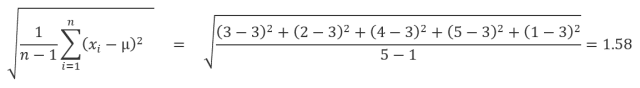The standard measure of uncertainty is the standard deviation so one ‘standard uncertainty‘ means one standard deviation in the measurement.

#### Probability Distribution

To understand what a probability distribution is, imagine rolling a six-sided die. You have an equal chance of rolling a 1, 2, 3, 4, 5 or 6. Imagine you roll the die 1,000 times and plot each score against the number of times you got it. Assuming the die is fair, your graph will have six bars of roughly equal height forming a rectangular shape known as a rectangular distribution.

The uncertainty due to rounding a measurement result to the nearest increment on an instrument’s scale has this rectangular—or uniform—distribution, since there is an equal chance of the true value being anywhere between +/- half an increment on either side.

If you roll 2 dice, your score can be between 2 and 12 but you are more likely to get a 7 than a 2 or a 12. If we label the dice A and B then there is only one way to score 2 (A=1 and B=1) or 12 (A=6 and B=6). But there are 2 ways to score 3 (A=1 and B=2) or (A=2 and B=1). What we see is a triangular distribution, with the most likely score being a 7.

Ways to score 2 : (1,1)

Ways to score 3 : (1,2)(2,1)

Ways to score 4 : (1,3)(2,2)(3,1)

Ways to score 5 : (1,4)(2,3)(3,2)(4,1)

Ways to score 6 : (1,5)(2,4)(3,3)(4,2)(5,1)

Ways to score 7 : (1,6)(2,5)(3,4)(4,3)(5,2)(6,1)

Ways to score 8 : (2,6)(3,5)(4,4)(5,3)(6,2)

Ways to score 9 : (3,6)(4,5)(5,4)(6,3)

Ways to score 10 : (4,6)(5,5)(6,4)

Ways to score 11 : (5,6)(6,5)

Ways to score 12 : (6,6)

This doesn’t only apply to dice: if you combine two random effects with uniform distributions of similar magnitude you get a triangular distribution.

As you add more dice (or other random effects) the peak of the triangle starts to flatten and the ends start to trail off to form a bell shape known as the Gaussian, or normal, distribution. If you think about this you could consider the Gaussian distribution to be made up of lots of uniform distributions or, equally, of lots of triangular distributions.

Actually, if you add together a sufficient number of distributions of any shape, you end up with a normal distribution. For this reason, combined uncertainty is usually assumed to be normally distributed even though the sources may not be.The Central Limit Theorem – as we add together distributions of any shape the combined distribution tends towards a normal distribution. (Image courtesy of the author.)
Assuming the combined uncertainty is normal allows us to estimate probabilities or confidence limits. For example, we can have 68 percent confidence that the true value is within one standard uncertainty of the measurement result and 95 percent confidence that it is within two standard uncertainties.

## Common Sources of Uncertainty

There are many sources of uncertainty. In almost any type of measurement there will be uncertainty associated with the reference standard used for calibration, the repeatability of the calibration process and the repeatability of the actual measurement. Environmental influences, such as temperature, are also often important.

For digital instruments, resolution or rounding may not be a significant source of uncertainty as they often read out to decimal places beyond their repeatability. For manually read gauges, such as rulers and dial gauges, the resolution or rounding is much more likely to be important.

The maximum possible error due to rounding is half of the resolution. For example, when measuring with a ruler which has a resolution of 1 mm, the rounding error will be +/- 0.5 mm with a uniform distribution.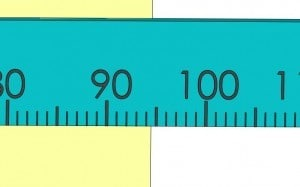Rounding errors can be up to half of the resolution of an instrument. (Image courtesy of the author.)
When an instrument is calibrated, it is compared to a reference standard. Error in the reference standard is therefore transferred to the instrument being calibrated, since this error is unknown: it is the uncertainty of the reference standard which in inherited by the instrument. The comparison process is also imperfect and introduces additional uncertainty.

Both of these sources will result in a systematic uncertainty which will not be detectable by a Type A evaluation. A calibration certificate produced by an accredited calibration lab must include a statement of this calibration uncertainty.

The value stated on the calibration certificate is often mistakenly taken as the uncertainty of measurements made using the instrument. This is wrong: it is only one source of uncertainty and measurements made using the instrument may have significantly higher uncertainty, which is why all sources of uncertainty must be considered and combined.

Repeatability is estimated by making a series of measurements, generally by the same person and under the same conditions, and then finding the standard deviation of these measurements. This is a Type A evaluation.

Reproducibility is estimated by making a series of measurements under changed conditions. The conditions which are changed are often the operator and the part being measured, according to a standard Gage R&R study. However, it’s important to consider which conditions are significant and which are evaluated in some other way.

Environmental effects, such as temperature, are often significant and sometimes the most challenging to evaluate. For example, thermal expansion creates linear expansion in both the object being measured and in the measuring instrument; these effects may partially but not completely cancel each other, and there may also be non-linear distortions caused by thermal expansion.

For dimensional measurement, alignment is often a significant source of uncertainty. For example, when a measurement of the distance between two parallel surfaces is made, the measurement should be perpendicular to the surfaces. Any angular deviation from the perpendicular measurement path will result in a cosine error, in which the actual distance is the measured distance multiplied by the cosine of the angular deviation.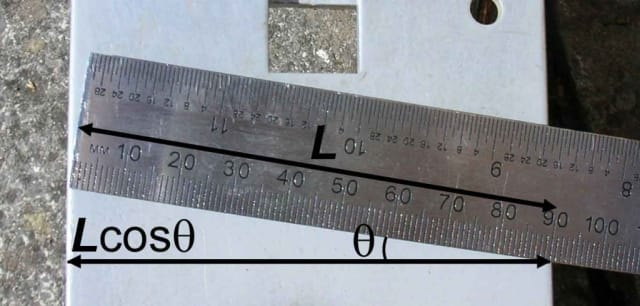Cosine error is a common source of uncertainty of measurement. (Image courtesy of the author.)

Abbe results from an offset between the axis along which an object is being measured and the axis of the instrument’s measurement scale—the distance between these axes is known as the Abbe Offset. If the distance moved along the object axis is not transferred in a perpendicular direction to the scale axis then the measurement is erroneous.

The Abbe error is the tangent of the angular offset multiplied by the Abbe Offset. Callipers are particularly susceptible to Abbe Error, since there is a significant Abbe offset between the scale and the object being measured. Micrometers were designed to minimize the Abbe offset and it is largely for this reason that they are significantly more accurate than callipers.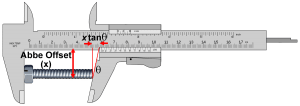Callipers are susceptible to Abbe Error because there is a significant offset between the measurement scale and the object being measured. (Image courtesy of the author.)

## Calculating a Combined Uncertainty

To calculate a combined uncertainty, you must first quantify the individual sources of uncertainty using either Type A or Type B estimates. Combining them to give the uncertainty of a measurement is not as simple as adding them together.

The probability that errors resulting from different sources will all be maximal or minimal at the same time is very small. This means that simply adding them would over-estimate the uncertainty. A statistical combination is therefore required. The law for the propagation of uncertainty provides a way to do this, and although the mathematics can be a bit intense, if we use an uncertainty budget, it’s not so bad.

An uncertainty budget is a table in which we list each source of uncertainty together with its value, distribution and sensitivity coefficient. We can then calculate standard uncertainties for each source and finally combine these.

This is best explained with an example.

Consider the uncertainty budget below, which lists three sources of uncertainty. The calibration uncertainty is taken from the calibration certificate, which shows a value of 3 µm. Because the calibration uncertainty is assumed to result from all the sources in combination, we assume that it is normally distributed.

The confidence value given on the certificate is 95 percent, so we divide by 2 to give a standard uncertainty. We multiply the value by the sensitivity coefficient and divide by the divisor to obtain a standard uncertainty.

For now, we will ignore the sensitivity coefficients and assume they are all equal to one. The resolution of the instrument means that results are rounded to the nearest 10 µm and so there is a rectangular distribution of +/- 5 µm.

To convert a rectangular distribution to a standard uncertainty we divide by —this is a standard factor which is used by metrologists. The repeatability is obtained by directly calculating the standard deviation of a number of measurements, the divisor is simply 1.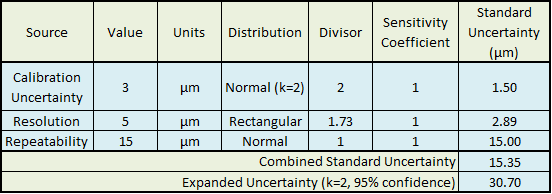To find the combined standard uncertainty we square each of the standard uncertainties for each source, sum the squared values and then find the square root of the sum. Like this:You now have a good idea of how to estimate the uncertainty for a measurement.

This method will work for cases where all your sources have the same units as your measurement result and have a direct proportional effect on the result, so the sensitivities are equal to one.

This would be true for the sources given in the example. For many cases it is not true.

In my next article, I will explain when this may not be the case and show you how to deal with those cases.Dr. Jody Muelaner’s 20-year engineering career began in machine design, working on everything from medical devices to saw mills. Since 2007 he has been developing novel metrology at the University of Bath, working closely with leading aerospace companies. This research is currently focused on uncertainty modelling of production systems, bringing together elements of SPC, MSA and metrology with novel numerical methods. He also has an interest in bicycle design. Visit his website for more information.